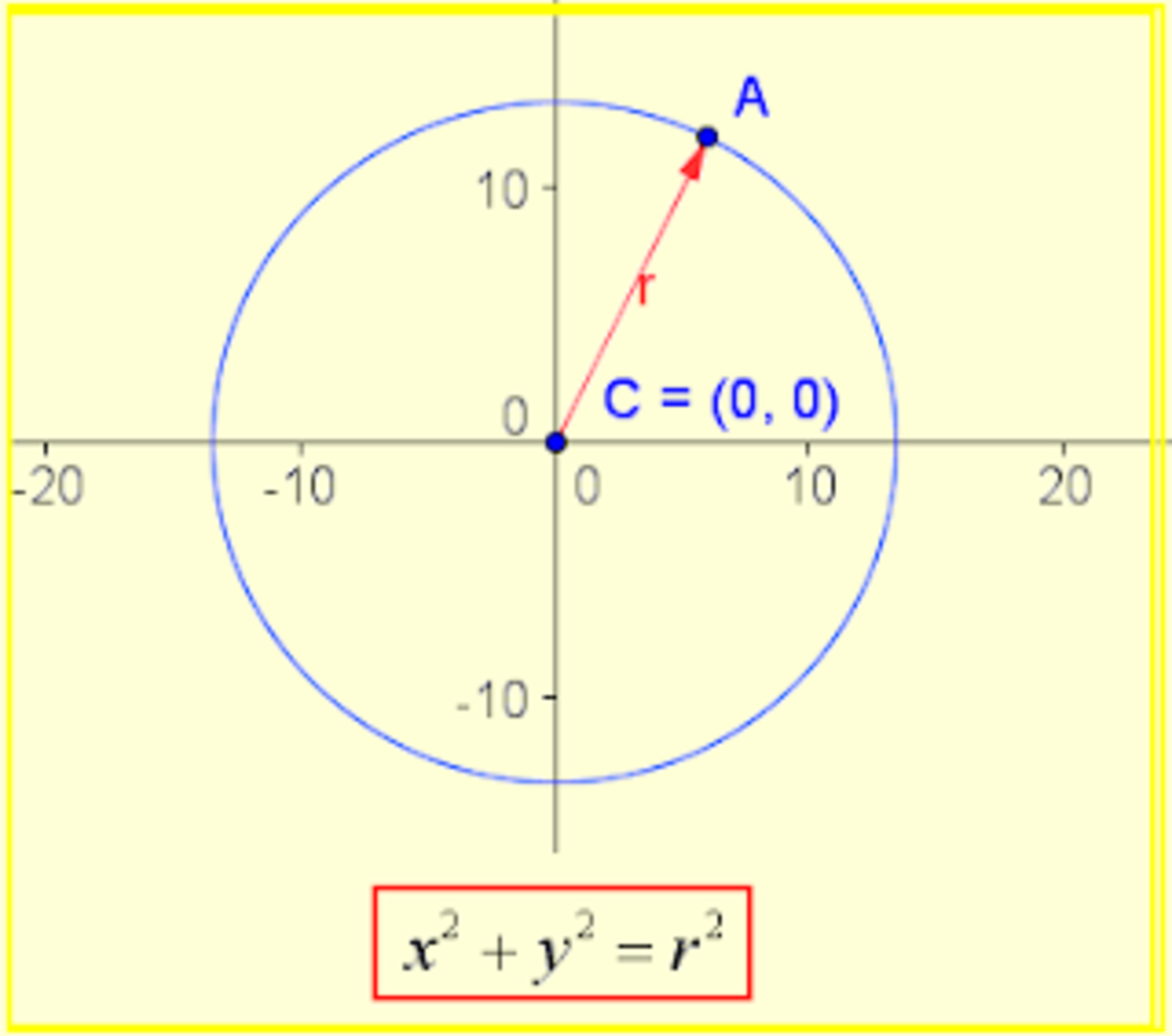# Math 6: Circumference and Intersection

A Biomedical science student, certificated in english, spanish, french and japanese language, who also writes diverse topic articles.

Content

• Circunference

• Elements of the circumference

• Equation of the circumference with center at (0,0)

• Equation of the circle with center (h, k)

• General equation of circumference

## Circumference

A circumference is the set of all the points of a plane that are equidistant from another fixed and coplanar point called the center.
The distance between any of its points and the center is called the radius. The line segment formed by two aligned radius is called the diameter, it is the greatest possible distance between two points that belong to the circumference and it's length is twice the length of the radius. It is distinguished from the circle in that this is the perimeter of the circle while the surface it contains is the circle.

It can be considered as an ellipse with zero eccentricity, or an ellipse whose semi-axes are equal. It can also be described as the perpendicular section to the axis of a conical or cylindrical surface, or as a polygon with infinite sides, whose apothem coincides with its radius.

## Elements of the circumference

There are several points, lines and segments in each circumference.

• Center: It is the interior point that has the same distance from all the points of the circumference.
• Radius: The segment that conects the center with any point on the circumference.
• Diameter: It is the largest segment that conects two points on the circumference (necessarily passes through the center).
• Chord: It is the segment that conects two points of the circumference without having to go through the center, the chords of maximum length are those that pass through the center and those are the diameter.
• Secant line: It is the one that cuts the circumference at two of its points.
• Tangent line: It is the one that touches the circumference in only one of its points.
• Point of tangency: It is that point of contact of the tangent line with the circumference.
• Arch: It is the curvilinear segment of points belonging to the circumference.
• Semicircle: It is that arc delimited by the ends of a diameter.

## Equation of the circumference with center at (0,0)

When the center of a circle is at the origin of a Cartesian plane (0, 0) the equation is said to be in its canonical form, that is, its form x2 + y2 = r2.The circumference with center at the origin and radius equal to 1 is called goniometric circumference or unit circumference.
Scroll to Continue

## Equation of the circle with center (h, k)

In a Cartesian coordinate system x - y, the circumference with center at the point (h, k) is that in which the center is different from the point of origin (0, 0), this equation is in its ordinary form, which is the form (x - h) ² + (y - k) ² = r², where (h, k) is the center and r is the radius.

## General equation of circumference

If we know the center and radius of a circumference, we can construct its ordinary equation and if we operate the squares, we get the general form of the equation of the circumference.

• (x - h)² + (y - k)² = r²
• x² - 2xh + h² + y² - 2yk + k² = r²
• x² - 2xh + h² + y² - 2yk + k² - r² = 0
• x² + y² - 2xh - 2yk + h² + k² - r² = 0

© 2021 Daniela Alejandra Rodríguez Cerda